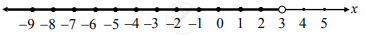# Sketch the graph which represents the solution set for the following inequation.x < 3 - Mathematics and Statistics

Graph

Sketch the graph which represents the solution set for the following inequation.
x < 3

#### Solution

x < 3
Here, x takes all real values that are less than 3.
∴ Solution set represents the unbounded (open) interval (−∞,3)
∴ the required graph of the solution set is as follows:Concept: Graphical Representation of Solution of Linear Inequality in One Variable
Is there an error in this question or solution?

#### APPEARS IN

Balbharati Mathematics and Statistics 2 (Commerce) 11th Standard HSC Maharashtra State Board
Chapter 8 Linear Inequations
Exercise 8.1 | Q 3. (iii) | Page 116